# Energy Stored in Capacitors Notes | Study Basic Physics for IIT JAM - Physics

## Physics: Energy Stored in Capacitors Notes | Study Basic Physics for IIT JAM - Physics

The document Energy Stored in Capacitors Notes | Study Basic Physics for IIT JAM - Physics is a part of the Physics Course Basic Physics for IIT JAM.
All you need of Physics at this link: Physics

Most of us have seen dramatizations in which medical personnel use a defibrillator to pass an electric current through a patient’s heart to get it to beat normally. Often realistic in detail, the person applying the shock directs another person to “make it 400 joules this time.” The energy delivered by the defibrillator is stored in a capacitor and can be adjusted to fit the situation. SI units of joules are often employed. Less dramatic is the use of capacitors in microelectronics, such as certain handheld calculators, to supply energy when batteries are charged. Capacitors are also used to supply energy for flash lamps on cameras.
Energy stored in the large capacitor is used to preserve the memory of an electronic calculator when its batteries are charged. (credit: Kucharek, Wikimedia Commons)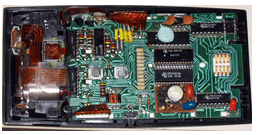Energy stored in a capacitor is electrical potential energy, and it is thus related to the charge Q and voltage V on the capacitor. We must be careful when applying the equation for electrical potential energy PE =qV to a capacitor. Remember that PE is the potential energy of a charge q going through a voltage V. But the capacitor starts with zero voltage and gradually comes up to its full voltage as it is charged. The first charge placed on a capacitor experiences a change in voltage V=0, since the capacitor has zero voltage when uncharged. The final charge placed on a capacitor experiences V=V, since the capacitor now has its full voltage V on it. The average voltage on the capacitor during the charging process is V/2, and so the average voltage experienced by the full charge q is V/2. Thus the energy stored in a capacitor, Ecap, is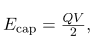where Q is the charge on a capacitor with a voltage V applied. (Note that the energy is not QV, but QV/2) Charge and voltage  are related to the capacitance C of a capacitor by Q=CV, and so the expression for Ecap can be algebraically manipulated into three equivalent expressions: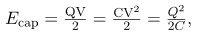where Q is the charge and V the voltage on a capacitor C. The energy is in joules for a charge in coulombs, voltage in volts, and capacitance in farads.

Energy Stored in Capacitors

The energy stored in a capacitor can be expressed in three ways: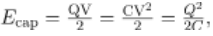where Q is the charge, V is the voltage, and C is the capacitance of the capacitor. The energy is in joules for a charge in coulombs, voltage in volts, and capacitance in farads.

In a defibrillator, the delivery of a large charge in a short burst to a set of paddles across a person’s chest can be a lifesaver. The person’s heart attack might have arisen from the onset of fast, irregular beating of the heart—cardiac or ventricular fibrillation. The application of a large shock of electrical energy can terminate the arrhythmia and allow the body’s pacemaker to resume normal patterns. Today it is common for ambulances to carry a defibrillator, which also uses an electrocardiogram to analyze the patient’s heartbeat pattern. Automated external defibrillators (AED) are found in many public places. These are designed to be used by lay persons. The device automatically diagnoses the patient’s heart condition and then applies the shock with appropriate energy and waveform. CPR is recommended in many cases before use of an AED.
Automated external defibrillators are found in many public places. These portable units provide verbal instructions for use in the important first few minutes for a person suffering a cardiac attack. (credit: Owain Davies, Wikimedia Commons)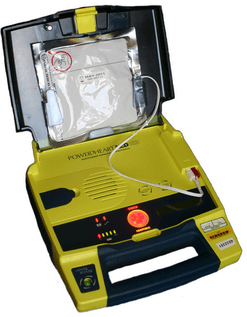Capacitance in a Heart Defibrillator
A heart defibrillator delivers 4.00102 J of energy by discharging a capacitor initially at 1.00104 V. What is its capacitance?

Strategy

We are given Ecap and V, and we are asked to find the capacitance C. Of the three expressions in the equation for Ecap, the most convenient relationship is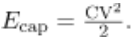Solution

Solving this expression for C and entering the given values yields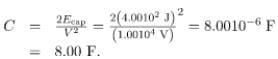Discussion

This is a fairly large, but manageable, capacitance at 1.00104  V.

Section Summary

• Capacitors are used in a variety of devices, including defibrillators, microelectronics such as calculators, and flash lamps, to supply energy.
• The energy stored in a capacitor can be expressed in three ways: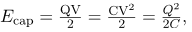where

Q is the charge, V is the voltage, and C is the capacitance of the capacitor. The energy is in joules when the charge is in coulombs, voltage is in volts, and capacitance is in farads.

Conceptual Questions

How does the energy contained in a charged capacitor change when a dielectric is inserted, assuming the capacitor is isolated and its charge is constant? Does this imply that work was done?
What happens to the energy stored in a capacitor connected to a battery when a dielectric is inserted? Was work done in the process?

Problems & Exercises

(a) What is the energy stored in the
10.0 F capacitor of a heart defibrillator charged to
9.00103 V? (b) Find the amount of stored charge.

(a) 405 J

(b) 90.0mC

In open heart surgery, a much smaller amount of energy will defibrillate the heart. (a) What voltage is applied to the 8.00 F capacitor of a heart defibrillator that stores 40.0 J of energy? (b) Find the amount of stored charge.

(a) 3.16 kV

(b) 25.3 mC

A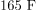capacitor is used in conjunction with a motor. How much energy is stored in it when 119 V is applied?

Suppose you have a 9.00 V battery, a
2.00 F
capacitor, and a 7.40 F capacitor. (a) Find the charge and energy stored if the capacitors are connected to the battery in series. (b) Do the same for a parallel connection.

(a)  1.42105 C,  6.38105 J

(b)  8.4610C, 3.81104

A nervous physicist worries that the two metal shelves of his wood frame bookcase might obtain a high voltage if charged by static electricity, perhaps produced by friction. (a) What is the capacitance of the empty shelves if they have area 1.00102 m2 and are 0.200 m apart? (b) What is the voltage between them if opposite charges of magnitude 2.00 nC are placed on them? (c) To show that this voltage poses a small hazard, calculate the energy stored.

(a) 4.4310-12 F

(b) 452 V

(c) 4.521-7 J

Show that for a given dielectric material the maximum energy a parallel plate capacitor can store is directly proportional to the volume of dielectric (Volume =Ad). Note that the applied voltage is limited by the dielectric strength.

Consider a heart defibrillator similar to that discussed. Construct a problem in which you examine the charge stored in the capacitor of a defibrillator as a function of stored energy. Among the things to be considered are the applied voltage and whether it should vary with energy to be delivered, the range of energies involved, and the capacitance of the defibrillator. You may also wish to consider the much smaller energy needed for defibrillation during open-heart surgery as a variation on this problem.

Unreasonable Results

(a) On a particular day, it takes 9.60103 J of electric energy to start a truck’s engine. Calculate the capacitance of a capacitor that could store that amount of energy at 12.0 V. (b) What is unreasonable about this result? (c) Which assumptions are responsible?

(a) 133 F

(b) Such a capacitor would be too large to carry with a truck. The size of the capacitor would be enormous.

(c) It is unreasonable to assume that a capacitor can store the amount of energy needed.

The document Energy Stored in Capacitors Notes | Study Basic Physics for IIT JAM - Physics is a part of the Physics Course Basic Physics for IIT JAM.
All you need of Physics at this link: PhysicsUse Code STAYHOME200 and get INR 200 additional OFF

## Basic Physics for IIT JAM

220 videos|156 docs|94 tests

Track your progress, build streaks, highlight & save important lessons and more!

,

,

,

,

,

,

,

,

,

,

,

,

,

,

,

,

,

,

,

,

,

;